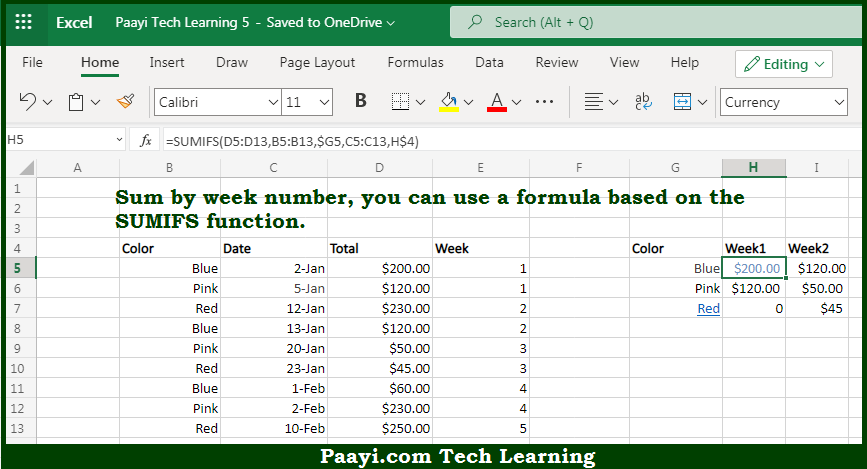# Learn How to SUM By Week Number in Microsoft Excel

Written by | 0 Comments | 612 Views

In this article, you will learn how to COUNT various things in Microsoft Excel using a single/combination(s) of functions. You will also know how to SUM By Week Number and see the generic formula.

SUM By Week Number in Microsoft Excel

The main purpose of this formula is to sum by week number. Here we will learn how to sum by provided week number in the workbook in Microsoft Excel. That implies, with the help of a formula based on the SUMIFS function you can able sum by week number. So, with the help of this formula, you can able to sum by provided the week number in the workbook in Microsoft Excel.

General Formula to SUM By Week Number

=SUMIFS(sumrange,weekrange,week)

The Explanation for the SUM By Week NumberSo we know that with the help of the given formula above you can able to sum by week number. Here we will learn how to sum by provided week number in the workbook in Microsoft Excel. As we know that the SUMIFS function is used to sum ranges based on multiple criteria. In the example given above, we configure SUMIFS to sum amounts in the named range total by week number utilizing two basic criteria - color = value in column G, and week = value in row 4. It should be noted that the \$G5 and H\$4 are both mixed references, so the formula can be copied across the table. So, with the help of this formula, you can able to sum by provided week in the workbook in Microsoft Excel.